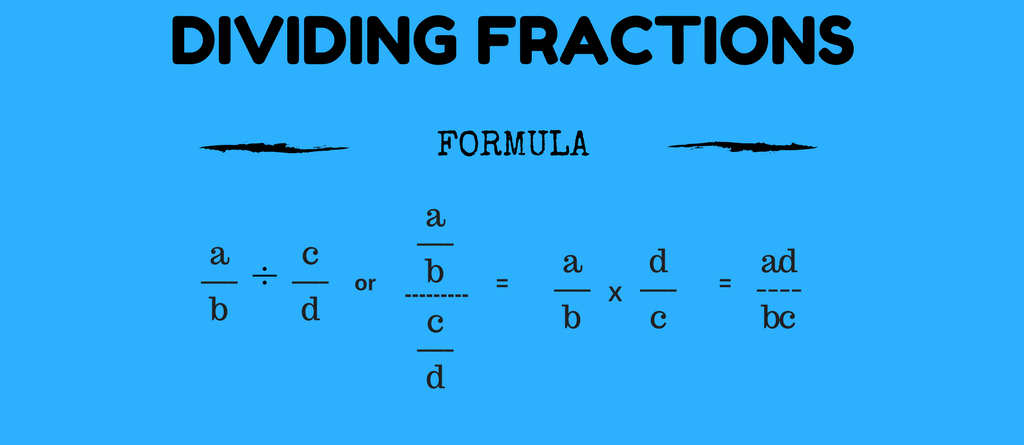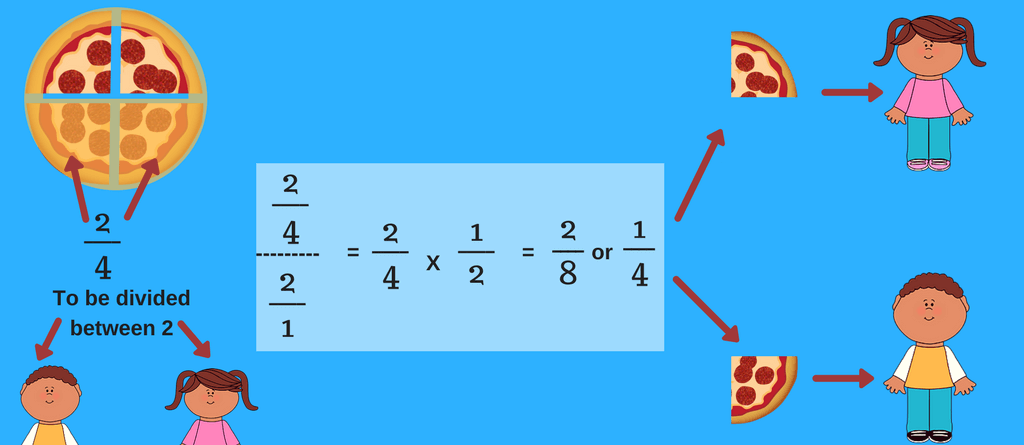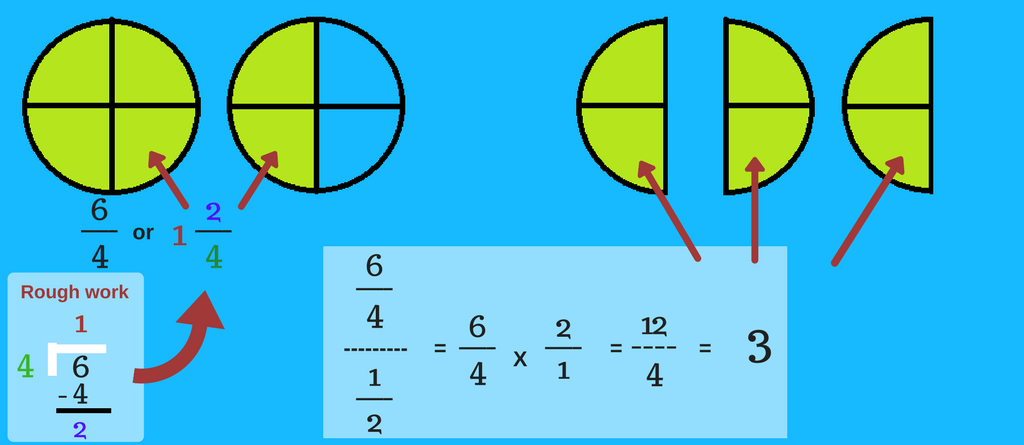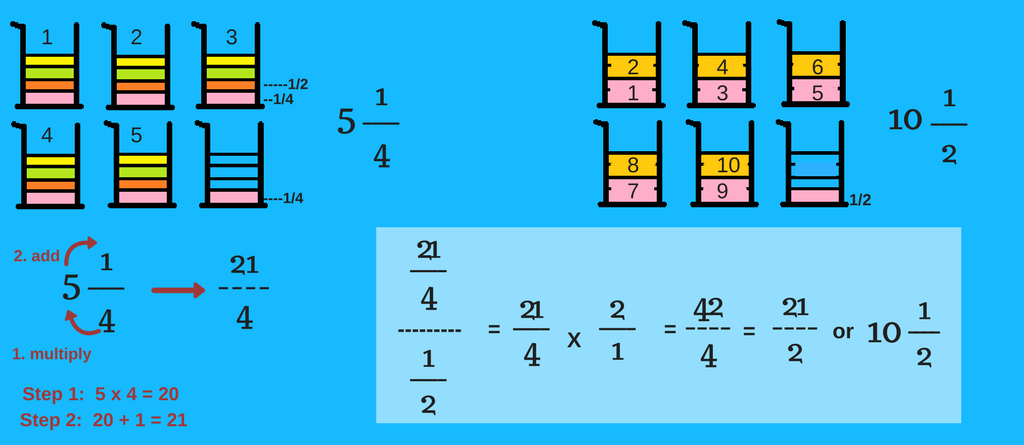Dividing fractions has been a monstrous task for kids with too many steps and technicalities involved in it. Well, now you don’t have to worry anymore because we explain and show theÂ simple steps involved in dividing fractions. Understand with examples and grab FREE math resource to get better practice. Yes, the whole bundle to learn dividing fractions is here. So, let’s divide!

How to divide fractions?

Formula for dividing fractionsLet’s understand this process of dividing fractions with examples.

1. Problem – 3/7 divided by 4/9

 3 Ã· 4 7 9

Step 1

Multiply the numerator of one fraction with the denominator of the other, it is as simple as that.

 3 x 9 = 27 7 x 4 28

Step 2

Now all you have to do it simply check if the resulting fraction can be further simplified in fraction form or not.

27/28Â is in its simplest fraction form.

How easy was that!

Now let’s try some more examples.

2. Problem – You have 2/4 of a pizza and you want to share it equally between 2 people. How much of the pizza does each person get?

Solution:

 2 Ã· 2 4 1

Step 1

Multiply the numerator of one fraction with the denominator of the other.

 2 x 1 = 2 4 x 2 8

Step 2

Simply.

 1 4

So, 1/4 pizza can be shared between 2 people.3. Problem – How many halves are there in six-fourth?

Solution:Â

 6 Ã· 1 4 2

Step 1

Multiply the numerator of one fraction with the denominator of the other.

 6 x 2 = 12 4 x 1 4

Step 2

Simply.

 3 1

Or 3. There are 3 halves in six-fourth.

How to convert 6/4 into mixed fraction? Easy. Divide 6 by 4. The remainder will be the numerator and the quotient will be the whole number (denominator is the same). So, 6 divided by 4, remainder is 2 and quotient is 1.4. Problem – How many 1/2 cup servings are in a jar of mixed juice that contains 5 1/4 cups altogether?

Solution:

Step 0 –

Convert the mixed fraction into improper fraction.

(4 x 5) + 1 = 21, resulting in

 21 4

Feel free to use mixed fraction calculator for quick conversion.

 21 Ã· 1 4 2

Step 1

Multiply the numerator of one fraction with the denominator of the other.

 21 x 2 = 42 4 x 1 4

Step 2

Now, simply.

 21 2

The answer – 21 / 2 or 10 1/2 cups of serving.

How to convert 21/2 into mixed fraction? Easy. Divide 21 by 2. The remainder will be the numerator and the quotient will be the whole number (denominator is the same). So, 21 divided by 2, remainder is 1 and quotient is 10.DividingÂ Fractions – More Resources

Free Online Fraction Calculator

Well, dividing fractionsÂ is actually too easy and quick. What’s quicker is the calculation of fractions in fraction calculator. The answer is provided with steps for better understanding. Try Fraction calculator now.

Free Math Worksheets

Fraction worksheets can give you fun practice experience. These math worksheets have story problems that helps you to relate to and understand the problem. Download math printable for freeÂ to practice dividing fractions and more.

Math Games

What’s better than play based learning? Â Make fraction practice fun and interesting while you play with Froggy Fraction and Monkey Fraction. It helps in learning, understanding, practicing, bonding and having fun while you play. (Also available on Amazon).

So, here’s hoping that this blog helped you with understanding dividingÂ fractions. If any doubts, do share with us. Use math resources to get better practice in a fun way. Â If you are confused with more fraction problems with different mathematical operation, try ‘understanding adding and subtracting fractions’ and ‘multiplying fractions‘. Here’s hoping that dividing fractions is no more of a monstrous task for you.